# Solutions to Time-Fractional Diffusion-Wave Equation in Cylindrical Coordinates

## Abstract

Nonaxisymmetric solutions to time-fractional diffusion-wave equation with a source term in cylindrical coordinates are obtained for an infinite medium. The solutions are found using the Laplace transform with respect to time, the Hankel transform with respect to the radial coordinate, the finite Fourier transform with respect to the angular coordinate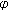, and the exponential Fourier transform with respect to the spatial coordinate. Numerical results are illustrated graphically.

## 1. Introduction

The time-fractional diffusion-wave equation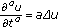(1.1)

is a mathematical model of important physical phenomena ranging from amorphous, colloid, glassy, and porous materials through fractals, percolation clusters, random, and disordered media to comb structures, dielectrics and semiconductors, polymers, and biological systems (see  and references therein).

The fundamental solution for the fractional diffusion-wave equation in one space-dimension was obtained by Mainardi . Wyss  obtained the solutions to the Cauchy problem in terms of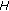-functions using the Mellin transform. Schneider and Wyss  converted the diffusion-wave equation with appropriate initial conditions into the integrodifferential equation and found the corresponding Green functions in terms of Fox functions. Fujita  treated integrodifferential equation which interpolates the diffusion equation and the wave equation. Hanyga  studied Green functions and propagator functions in one, two, and three dimensions.

Previously, in studies concerning time-fractional diffusion-wave equation in cylindrical coordinates, only one or two spatial coordinates have been considered . In this paper, we investigate solutions to (1.1) in an infinite medium in cylindrical coordinates in the case of three spatial coordinates,, and.

## 2. Statement of the Problem

Consider the time-fractional diffusion-wave equation with a source term in cylindrical coordinates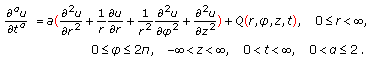(2.1)

The initial conditions are prescribed: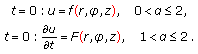(2.2)

In (2.1), we use the Caputo fractional derivative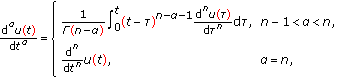(2.3)

where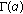is the gamma function. For its Laplace transform rule, the Caputo fractional derivative requires the knowledge of the initial values of the function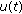and its integer derivatives of order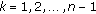: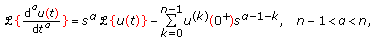(2.4)

whereis the transform variable.

The solution to the initial-value problem (2.1)-(2.2) can be written in the following form:(2.5)

Now, we investigate the fundamental solutions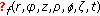,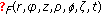, and.

## 3. Fundamental Solution to the First Cauchy Problem

In the case of the first Cauchy problem, the initial value of a sought-for function is prescribed. Hence,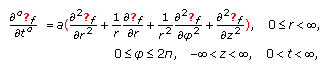(3.1)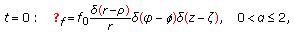(3.2)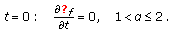(3.3)

The two-dimensional Dirac delta function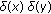after passing to the polar coordinates takes the form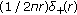, but for the sake of simplicity, we have omitted the multiplier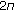in the solution (2.5) as well as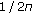in (3.2). In the initial condition (3.2), we have introduced the constant multiplier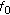to obtain the nondimensional quantity(see (3.10)).

The solution is found using the Laplace transform with respect to time, the Hankel transform with respect to the radial coordinate, the finite Fourier transform with respect to the angular coordinate, and the exponential Fourier transform with respect to the spatial coordinate. In the transforms domain we get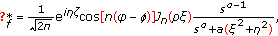(3.4)

where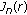is the Bessel function of the first kind of order, the asterisk indicates the transforms,is the Laplace transform variable,is the Hankel transform variable,is exponential Fourier transform variable, and the integeris finite Fourier transform variable.

Inversion of integral transforms gives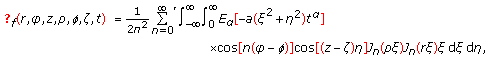(3.5)

where the prime denotes that the term corresponding to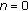in the sum should be multiplied by 1/2. In (3.5),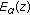is the Mittag-Leffler function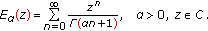(3.6)

The essential role of the Mittag-Leffler function in fractional calculus results from the following formula for the inverse Laplace transform :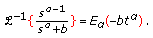(3.7)

If the solution does not depend on the coordinate, then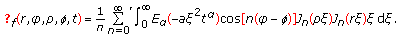(3.8)

The fundamental solution (3.8) was considered in  for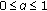.

In the case when the solution does not also depend on the angular coordinate, we get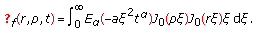(3.9)

Dependence of fundamental solution (3.5) on the coordinates,, andis presented in Figures 1, 2, 3, and 4.

In calculations, we have introduced nondimensional quantities: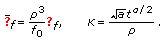(3.10)

## 4. Fundamental Solution to the Second Cauchy Problem

In the case of the second Cauchy problem, the initial value of the time derivative of a sought-for function is prescribed, and for the corresponding fundamental solution we have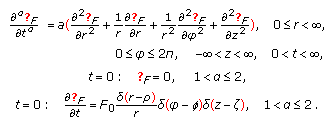(4.1)

In this instance, the fundamental solution is expressed as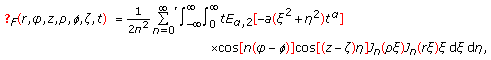(4.2)

where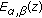is the generalized Mittag-Leffler function in two parametersand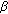: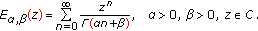(4.3)

We have used the following formula for the inverse Laplace transform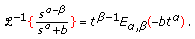(4.4)

It is evident that (3.7) is the particular case of (4.4) corresponding to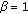.

If the solution does not depend on the coordinate, then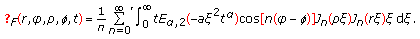(4.5)

In the case of axial symmetry ,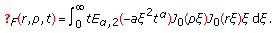(4.6)

Figures 5, 6, 7, and 8 show dependence of fundamental solution (4.2) on coordinates,, and, where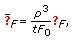(4.7)

and the nondimensional quantityis the same as in (3.10).

## 5. Fundamental Solution to the Source Problem

Consider the time-fractional diffusion-wave equation with a source term under zero initial conditions: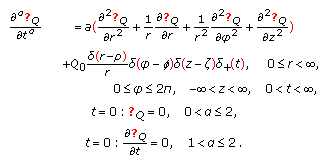(5.1)

The solution is obtained using the integral transform technique and reads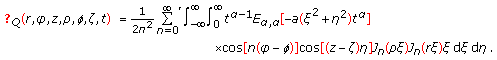(5.2)

If dependence of solution on the coordinateis not taken into account, then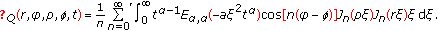(5.3)

In the case of axial symmetry ,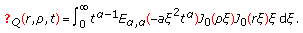(5.4)

Dependence of the solution (5.2) on the coordinates,, andis depicted in Figures 9, 10, and 11 with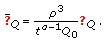(5.5)

## 6. Discussion

The solutions to the Cauchy and source problems for time-fractional diffusion-wave equation have been found in cylindrical coordinates. The considered equation in the case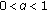interpolates the Helmholtz and diffusion equation. In the case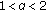, the time-fractional diffusion-wave equation interpolates the standard diffusion equation and the classical wave equation.

For, the solutions to the fractional diffusion-wave equation feature propagating humps, underlining the proximity to the standard wave equation in contrast to the shape of curves describing the subdiffusion regime ().

For better understanding of behavior of solutions, it is worthwhile to compare the obtained results with those for delta pulse applied at the origin investigated in . The Mittag-Leffler functions arising in (3.5), (4.2), and (5.2) for large values of argument are represented as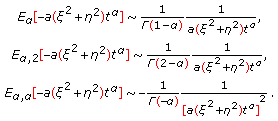(6.1)

Such asymptotic results in singularities of the solution to the first and the second Cauchy problems at the point of application of the delta pulse, whereas the solution to the source problem does not have singularity. Dependence of the solution on the angular coordinateat some distance from the point of the delta pulse application (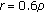in Figures 3 and 7) features only humps with no singularity.

## References

1. 1.

Mainardi F: Fractional calculus: some basic problems in continuum and statistical mechanics. In Fractals and Fractional Calculus in Continuum Mechanics (Udine, 1996), CISM Courses and Lectures. Volume 378. Edited by: Carpinteri A, Mainardi F. Springer, Vienna, Austria; 1997:291-348.

2. 2.

Pękalski A, Sznajd-Weron K (Eds): Anomalous Diffusion: From Basics to Applications. Springer, Berlin, Germany; 1999.

3. 3.

Metzler R, Klafter J: The random walk's guide to anomalous diffusion: a fractional dynamics approach. Physics Reports 2000,339(1):1-77. 10.1016/S0370-1573(00)00070-3

4. 4.

Zaslavsky GM: Chaos, fractional kinetics, and anomalous transport. Physics Reports 2002,371(6):461-580. 10.1016/S0370-1573(02)00331-9

5. 5.

West BJ, Bologna M, Grigolini P: Physics of Fractal Operators, Institute for Nonlinear Science. Springer, New York, NY, USA; 2003:x+354.

6. 6.

Metzler R, Klafter J: The restaurant at the end of the random walk: recent developments in the description of anomalous transport by fractional dynamics. Journal of Physics A 2004,37(31):R161-R208. 10.1088/0305-4470/37/31/R01

7. 7.

Povstenko YZ: Fractional heat conduction equation and associated thermal stress. Journal of Thermal Stresses 2005,28(1):83-102.

8. 8.

Magin RL: Fractional Calculus in Bioengineering. Begell House Publishers, Connecticut, Mass, USA; 2006.

9. 9.

Gafiychuk VV, Datsko BYo: Pattern formation in a fractional reaction-diffusion system. Physica A 2006,365(2):300-306. 10.1016/j.physa.2005.09.046

10. 10.

Uchaikin VV: Method of Fractional Derivatives. Artishock, Ulyanovsk, Russia; 2008.

11. 11.

Mainardi F: The fundamental solutions for the fractional diffusion-wave equation. Applied Mathematics Letters 1996,9(6):23-28. 10.1016/0893-9659(96)00089-4

12. 12.

Wyss W: The fractional diffusion equation. Journal of Mathematical Physics 1986,27(11):2782-2785. 10.1063/1.527251

13. 13.

Schneider WR, Wyss W: Fractional diffusion and wave equations. Journal of Mathematical Physics 1989,30(1):134-144. 10.1063/1.528578

14. 14.

Fujita Y: Integrodifferential equation which interpolates the heat equation and the wave equation. Osaka Journal of Mathematics 1990,27(2):309-321.

15. 15.

Hanyga A: Multidimensional solutions of time-fractional diffusion-wave equations. The Royal Society of London. Proceedings. Series A 2002,458(2020):933-957. 10.1098/rspa.2001.0904

16. 16.

Povstenko YZ: Stresses exerted by a source of diffusion in a case of a non-parabolic diffusion equation. International Journal of Engineering Science 2005,43(11-12):977-991. 10.1016/j.ijengsci.2005.03.004

17. 17.

Povstenko YZ: Two-dimensional axisymmetric stresses exerted by instantaneous pulses and sources of diffusion in an infinite space in a case of time-fractional diffusion equation. International Journal of Solids and Structures 2007,44(7-8):2324-2348. 10.1016/j.ijsolstr.2006.07.008

18. 18.

Achar BNN, Hanneken JW: Fractional radial diffusion in a cylinder. Journal of Molecular Liquids 2004,114(1-3):147-151. 10.1016/j.molliq.2004.02.012

19. 19.

Povstenko YZ: Fractional radial diffusion in a cylinder. Journal of Molecular Liquids 2008,137(1-3):46-50. 10.1016/j.molliq.2007.03.006

20. 20.

Özdemir N, Karadeniz D: Fractional diffusion-wave problem in cylindrical coordinates. Physics Letters A 2008,372(38):5968-5972. 10.1016/j.physleta.2008.07.054

21. 21.

Özdemir N, Agrawal OP, Karadeniz D, Iskender BB: Axis-symmetric fractional diffusion-wave problem: part I—analysis. Proceedings of the 6th Euromech Nonlinear Dynamics Conference (ENOC '08), June-July 2008, Saint Petrsburg, Russia

22. 22.

Lenzi EK, da Silva LR, Silva AT, Evangelista LR, Lenzi MK: Some results for a fractional diffusion equation with radial symmetry in a confined region. Physica A 2009,388(6):806-810. 10.1016/j.physa.2008.11.030

23. 23.

Özdemir N, Karadeniz D, İskender BB: Fractional optimal control problem of a distributed system in cylindrical coordinates. Physics Letters A 2009,373(2):221-226. 10.1016/j.physleta.2008.11.019

24. 24.

Özdemir N, Agrawal OP, Karadeniz D, Iskender BB: Fractional optimal control problem of an axis-symmetric diffusion-wave propagation. Physica Scripta T 2009, 136:-5.

25. 25.

Jiang X, Xu M: The time fractional heat conduction equation in the general orthogonal curvilinear coordinate and the cylindrical coordinate systems. Physica A 2010,389(17):3368-3374. 10.1016/j.physa.2010.04.023

26. 26.

Lenzi EK, Rossato R, Lenzi MK, da Silva LR, Gonçalves G: Fractional diffusion equation and external forces: solutions in a confined region. Zeitschrift für Naturforschung Section A 2010,65(5):423-430.

27. 27.

Qi H, Liu J: Time-fractional radial diffusion in hollow geometries. Meccanica 2010,45(4):577-583. 10.1007/s11012-009-9275-2

28. 28.

Gorenflo R, Mainardi F: Fractional calculus: integral and differential equations of fractional order. In Fractals and Fractional Calculus in Continuum Mechanics (Udine, 1996), CISM Courses and Lectures. Volume 378. Edited by: Carpinteri A, Mainardi F. Springer, Vienna, Austria; 1997:223-276.

29. 29.

Kilbas AA, Srivastava HM, Trujillo JJ: Theory and Applications of Fractional Differential Equations, North-Holland Mathematics Studies. Volume 204. Elsevier Science B.V., Amsterdam, The Netherlands; 2006:xvi+523.

30. 30.

Podlubny I: Fractional Differential Equations, Mathematics in Science and Engineering. Volume 198. Academic Press, San Diego, Calif, USA; 1999:xxiv+340.

31. 31.

Erdélyi A, Magnus W, Oberhettinger F, Tricomi FG: Higher Transcendental Functions. Vol. III. McGraw-Hill, New York, NY, USA; 1955:xvii+292.

32. 32.

Povstenko YZ: Fundamental solutions to three-dimensional diffusion-wave equation and associated diffusive stresses. Chaos, Solitons & Fractals 2008,36(4):961-972. 10.1016/j.chaos.2006.07.031

## Author information

Authors

### Corresponding author

Correspondence to Y.Z. Povstenko.

## Rights and permissions

Reprints and Permissions

Povstenko, Y. Solutions to Time-Fractional Diffusion-Wave Equation in Cylindrical Coordinates. Adv Differ Equ 2011, 930297 (2011). https://doi.org/10.1155/2011/930297